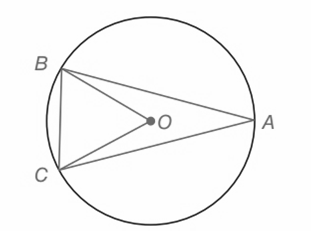Chapter 6.CT, Problem 2CTElementary Geometry For College St...

7th Edition
Alexander + 2 others
ISBN: 9781337614085

Solutions

Chapter
SectionElementary Geometry For College St...

7th Edition
Alexander + 2 others
ISBN: 9781337614085
Textbook Problem

a) If m B C ⌢ = 69 ° , then m ∠ B O C = _____________.b) If m B C ⌢ = 64 ° , then m ∠ B A C = _______________.To determine

(a)

To find:

The measure of mBOC

Explanation

Given:

mBC=69°

Approach:

1) In a circle the measure of a central angle is equal to the measure of the arc that it intercepts.

2) In a circle the measure of an interior angle is equal to one-half of the arc that it intercepts.

Calculation:

Consider the given circle,

mBC=69°.

BC is the arc that intercept the central angle

To determine

(b)

To find:

The measure of mBAC

Still sussing out bartleby?

Check out a sample textbook solution.

See a sample solution

The Solution to Your Study Problems

Bartleby provides explanations to thousands of textbook problems written by our experts, many with advanced degrees!

Get Started

Fill in each blank: 120ft=yd

Elementary Technical Mathematics

11. Maximize subject to

Mathematical Applications for the Management, Life, and Social Sciences

True or False: converges.

Study Guide for Stewart's Multivariable Calculus, 8th